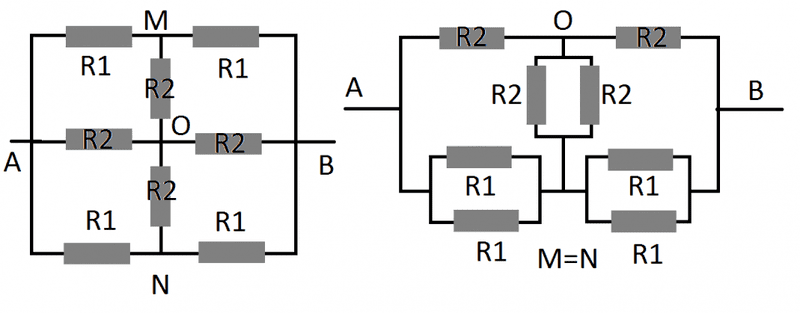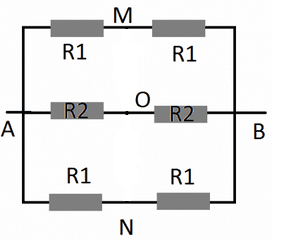# Equivalent electric circuit

Given an electric circuit, with resistors R1 and R2, find the equivalent resistance R.
Data: R1 = √2.R2

Points M and N have the same electric potential so they can be connected with a conductor and this way we get the second circuit.## Answers and Replies

mfb
Mentor
Points M and N have the same electric potential so they can be connected with a conductor and this way we get the second circuit.
There is more you can do to simplify the circuit.

In terms of what? I know i can solve the parallel ones below, however that won't solve it. There should be a way to proof that M and N are also equal to O.

mfb
Mentor
Sure, use symmetry again.

Sure, use symmetry again.
It's symmetrical along MO, NO but that doesn't help us much.

gneill
Mentor
Temporarily remove the two vertical R2's. What are the potentials at M,N,O?

Temporarily remove the two vertical R2's. What are the potentials at M,N,O?
On the upper side we will have a resistor with √2R2, but on the down side R2/√2. So they will be different.

gneill
Mentor
On the upper side we will have a resistor with √2R2, but on the down side R2/√2. So they will be different.
Really? What was your argument for M and N having the same potential in your first post?

Really? What was your argument for M and N having the same potential in your first post?
Equal amounts of charge will flow through the upper or downer R1, and since the resistance is equal the voltage will be too. However I don't understand why the points O and M or N will have the same potential as the resistance along the path is different.

gneill
Mentor
Equal amounts of charge will flow through the upper or downer R1, and since the resistance is equal the voltage will be too. However I don't understand why the points O and M or N will have the same potential as the resistance along the path is different.

The potentials at M,N, and O are due to the voltage dividers comprised of the resistances in their branches. Here's the situation:All three branches have the same potential difference across them (whatever you imagine to be placed across terminals AB). All three branches have the same resistance ratios...